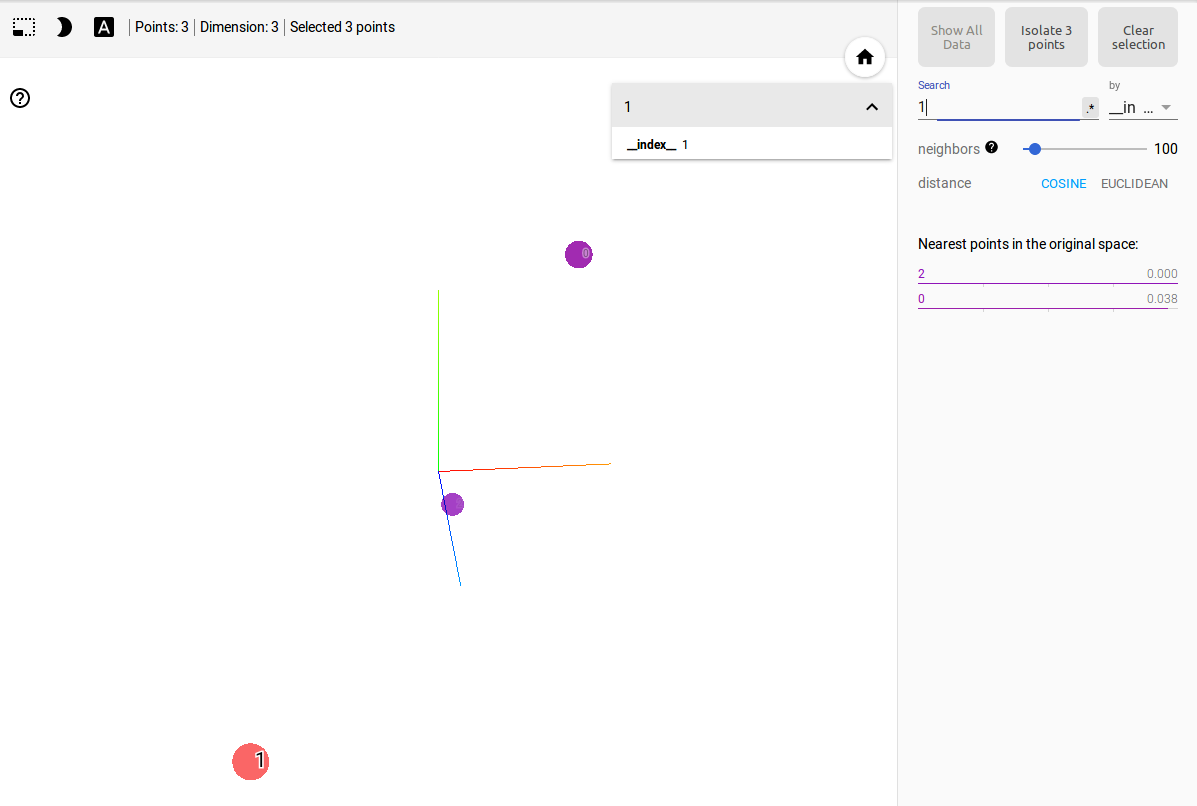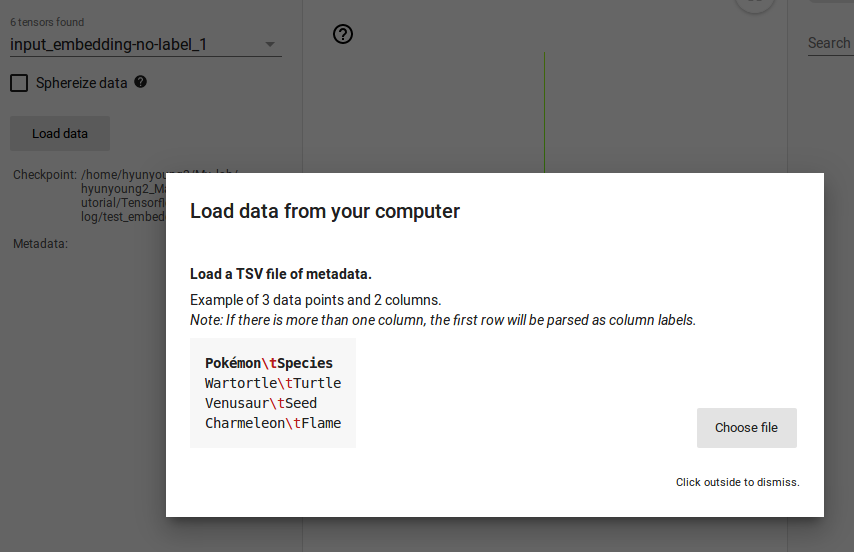# What is the word embedding?

An Embedding is a mapping from discrete object into vectors of real numbers. It is important to use text on task of Natural lanugauges processing, in particular, Machine learning and Deep Learning. For an example, When you embed words into vector space, It looks as follows:

``````blue:  (0.01359, 0.00075997, 0.24608, ..., -0.2524, 1.0048, 0.06259)
blues:  (0.01396, 0.11887, -0.48963, ..., 0.033483, -0.10007, 0.1158)
orange:  (-0.24776, -0.12359, 0.20986, ..., 0.079717, 0.23865, -0.014213)
oranges:  (-0.35609, 0.21854, 0.080944, ..., -0.35413, 0.38511, -0.070976)
``````

In case of vetor of word embedding, Specifically, We don’t know what each dimension in a vector means. However, We can realize some features from overall pattern of location and distance between vectors that Machine Learning take advantage of.

Because words of text can be represented as natural vectors,the way of word embedding is standard and effective way to transform such discrete. depending on how dense it is, and how better it utilize on the task that need vector of words of text.

# How to embed word in Tensorflow

To create word embeddings in Tensorflow, we first split the text into words and then we assign integer number to every word in every vocabulary as follow

``````text = "I have a cat."
# array has Index of each words.The Index is word_ids
# Index:  0,  1  , 2,  3 , 4  --> word_ids
# Array: [I, have, a, cat, .]
array_text = [I, have, a, cat, .]
``````

As you can see above, We need to map word_ids to vectors in Tensorflow. For this situation, use the following functions :

``````word_embeddings = tf.get_variable("word_embeddings", [vocabulary_size, embedding_size])
embedded_word_ids = tf.nn.embedding_lookup(word_embeddings, word_ids)
``````

The variable of word_embeddings is a array that is comprised of total vocabulary size and the dimesional size of each words we called embedding size. For example.

• a sentence(a document) : I have a cat.
• The total size of vocabularies : 5(I, have, a, cat, .)
• The size of embedding : whatever size you want, 300-dimensional for each words that normally used.

In detail, Let’s walk through the function of tf.nn.embedding_lookup.

``````word_embeddings = tf.get_variable("word_embeddings", [vocabulary_size, embedding_size])
embedded_word_ids = tf.nn.embedding_lookup(word_embeddings, word_ids)
``````

This function, tf.nn.embedding_lookup, literally means lookup in embeddings array depending on word_ids.

let’s say, we have word_emedddings matrix like this:

``````# word embedding function of tensorflow
# word_embeddings is 2 by 2 matrix, each factor is randomized.
# [[00, 01],
#  [10, 11]]
word_embeddings = tf.get_variable("word_embeddings_test", [2, 2])
# In here, 1 mean the first row of word_embedding matrix.
embedded_word_ids = tf.nn.embedding_lookup(word_embeddings, ids=1)

init = tf.global_variables_initializer()

with tf.Session() as sess:
sess.run(init)
embedding_result_test, embedded_word_result_test = sess.run([word_embeddings, embedded_word_ids])
print("=== the test result ===\n")
print("The result of word_embedding:\n",embedding_result_test, "\n")
print("The result of embedded_word_ids:\n",embedded_word_result_test, "\n")
``````

If you execute the graph above, the result of tf.nn.embedding_lookup(matrix, ids) is rows equal to ids. Let’s say ids set 1 as ids. the 1 index row of word_embeddings matrix is returned. If you enter ids such as a list([0, 1]), the return value is a list including row value of word_embeddings matrix depending the factors of list.

• If ids is 1, the return value is word_embedding

• If ids is [0, 1], the return value is [word_embedding, word_embedding]

Let’s see the result of computation graph above:

``````=== the test result ===

The result of word_embedding:
[[-0.7246539   0.43106437]
[ 0.52070427  1.06491148]]

The result of embedded_word_ids:
[ 0.52070427  1.06491148]
``````

# How to use Tensorflow projector as debugging.

When you are embedding text or image with Tensorflow, Tensorflow provide great tool to help you easily debug. It is calle Tensorboard.

Tensorboard is great tool. that draws your graph of computation and help you check some value of your model like FeedForward Neural Network. But, here you walk through how to project embedding matrix into 2-D ro 3-D.

let’s see an simple example code:

First is no label

``````# How to use tensorboard for WordEmbedding.
import os
import tensorflow as tf

LOG_DIR = os.path.join(os.getcwd(),"log/")
EMBEDDING_SIZE = 3
VOCA_SIZE = 3

print("the current working directory:", LOG_DIR, "\n")

# word vector of embedding.
embedding_input = tf.Variable([[0.1,0.2, 0.2],[0.5, 0.5, 0.5],[0.3,0.3,0.3]], dtype=tf.float32, name="input_embedding-no-label")

# For input of Tensorboard Projector
embeddings = tf.Variable(tf.zeros([VOCA_SIZE, EMBEDDING_SIZE], name="embedding_intial_tensor"), name="real_Embedding")
assignment = embeddings.assign(embedding_input)

# To add ops to save and restore all the variable
# When you want to make it
saver = tf.train.Saver()

# To initilize the variable.
global_init_op = tf.global_variables_initializer()

with tf.Session() as sess:
writer = tf.summary.FileWriter(LOG_DIR, sess.graph)
# To initialize all the variable in the default graph
sess.run(global_init_op)

# print embedding_input
embedding_input_, embeddings_ = sess.run([embedding_input, embeddings])
print("embedding_ipnut:\n", embedding_input_)
print("embeddings:\n", embeddings_)
assignment_, embeddings2_ = sess.run([assignment, embeddings])
print("assignment:\n", assignment_)
print("embeddings2:\n", embeddings2_)

save_path1 = saver.save(sess, os.path.join(LOG_DIR, "test_embedding_model.ckpt"), global_step=0)
print("\nModel Saved in file: %s" % save_path1)

writer.close()
``````

In here, you have to save checkpoint file to save all the variables on your model. if you completely save checkpoint file, move to directory you saved checkpoint file to.

and then prompt in command line like this:

tensorboard –logdir=the-path-you-saved-checkpoint-file-to

the result of the above code is:As you can see the code, there is no label for each words. so on Tensorboard Projector, each words are represented as index number.

Let’s say another one using with lable of embedding. In particular, here I tested it for NLP.

``````# How to use tensorboard for WordEmbedding.
import os
import tensorflow as tf

LOG_DIR = os.path.join(os.getcwd(),"log/")
EMBEDDING_SIZE = 3
VOCA_SIZE = 3

print("the current working directory:", LOG_DIR, "\n")

LABEL_FILE = os.path.join(os.getcwd(), "log/lables.tsv")

print("the current LABEL_FILE:", LABEL_FILE, "\n")

LABEL_NUM = 3
LABEL = ["NLP", "Deep Learning", "test"]

# Write label file
with open(LABEL_FILE,"w") as f:
for i in range(LABEL_NUM):
f.write(LABEL[i]+"\n")
print("labels.tsv file Created!\n")

# word vector of embedding.
embedding_input = tf.Variable([[0.1,0.2, 0.2],[0.5, 0.5, 0.5],[0.3,0.3,0.3]], dtype=tf.float32, name="input_embedding-no-label")

# For input of Tensorboard Projector
embeddings = tf.Variable(tf.zeros([VOCA_SIZE,EMBEDDING_SIZE], name="embedding_intial_tensor"), name="real_Embedding")
assignment = embeddings.assign(embedding_input)

# To initilize the variable.
init = tf.global_variables_initializer()

# To add ops to save and restore all the variable
# When you want to make it
saver = tf.train.Saver()

with tf.Session() as sess:

sess.run(init)

# print embedding_input
embedding_input_, embeddings_ = sess.run([embedding_input, embeddings])
print("embedding_ipnut:\n", embedding_input_)
print("embeddings:\n", embeddings_)
assignment_, embeddings2_ = sess.run([assignment, embeddings])
print("assignment:\n", assignment_)
print("embeddings2:\n", embeddings2_)

# Format: tensorflow/contrib/tensorboard/plugins/projector/projector_config.proto
config = tf.contrib.tensorboard.plugins.projector.ProjectorConfig()
embedding_config.tensor_name = embeddings.name

# Use the same LOG_DIR where you stored your checkpoint.
summary_writer = tf.summary.FileWriter(LOG_DIR)

# The next line writes a projector_config.pbtxt in the LOG_DtenIR. TensorBoard will
# read this file during startup.
tf.contrib.tensorboard.plugins.projector.visualize_embeddings(summary_writer, config)

save_path1 = saver.save(sess, os.path.join(LOG_DIR, "test_embedding_model.ckpt"), global_step=1)
print("\nModel Saved in file: %s" % save_path1)
``````

The different one from the former one is tf.contrib.tensorboard.plugins.projector.ProjectorConfig().

you have to set labal file(TSV file) up to Its return value(config)

If you create label file, the format of the file isto write words line by line depending on word_embedding matrix like this:

``````NLP\n
Deep Lerning\n
``````In order to use Tensorboard’s embedding projector, First you need variable to represent embedding data like embedding_temp on the above codes. And then just save checkpoint file to save all the variable of your model.

It is all what you have to do for projector of embeddin onto Tensorboard.

If you save checkpoint file, run the following:

tensorboard –logdir=the-path-you-saved-checkpoint-file-to

# To sum up

To visualize your embeddings, there are 3 things your need to do:

1) Set up a 2-D tensor variable(s) that holds your embedding(s):

embedding_var = tf.Variable(vocab_size, embedding_dimension)

2) Periodically save your embeddings in a LOG_DIR which is you want to save for checkpoint file.

saver = tf.train.Saver()
saver.save(session, op.path.join(LOG_DIR, “model.ckpt”), step)

The following step is now required , however if you have any metadata(labels, images) associated with your embedding, you need to link them to the tensor so TensorBoard knows about it.

If you have any metadata (labels, images) associated with your embedding, you can tell TensorBoard about it either by directly storing a projector_config.pbtxt in the LOG_DIR, or use our python API.

For instance, the following projector_config.ptxt associates the word_embedding tensor with metadata stored in \$LOG_DIR/metadata.tsv:

embeddings {
tensor_name: ‘word_embedding’
}

The same config can be produced programmatically using the following code snippet:

``````from tensorflow.contrib.tensorboard.plugins import projector
# Use the same LOG_DIR where you stored your checkpoint.
summary_writer = tf.train.SummaryWriter(LOG_DIR)

# Format: tensorflow/contrib/tensorboard/plugins/projector/projector_config.proto
config = projector.ProjectorConfig()

embedding.tensor_name = embedding_var.name

# Saves a configuration file that TensorBoard will read during startup.
projector.visualize_embeddings(summary_writer, config)
``````

You don’t need to write this Python code in your model, if you have metadata file(TSV.file), just on Tensorboard Embedding projector, load the metadata like this:If you are done with 3 processes above, at leat 2 processes except for metadata. After running your model and training your embeddings, run TensorBoard and point it to the LOG_DIR of the job

tensorboard –logdir=LOG_DIR

If you want to check an executed example code above, visit 01.Word_Embedding of hyunyoung2 git repository